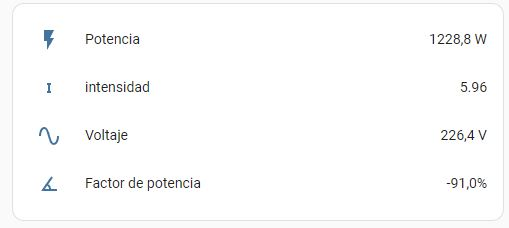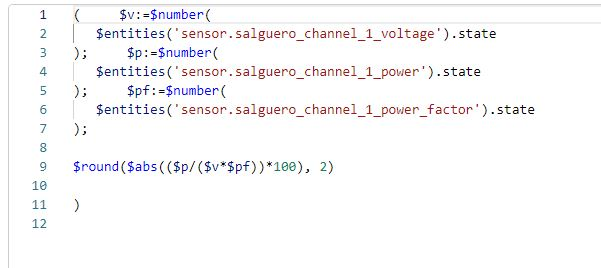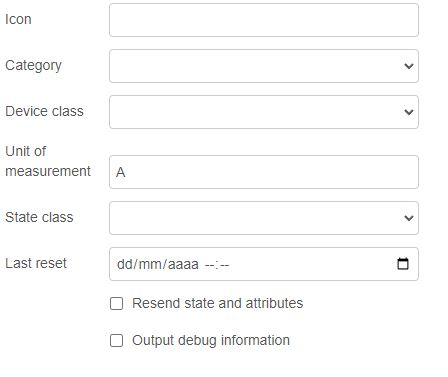# Mathematical formula to know the intensity (shelly em)

Hi,

I would like to know the intensity of my shelly using the formula I= P/(V*FP)

Entitys:
P = sensor.salguero_channel_1_power
V= sensor.salguero_channel_1_voltage
FP= sensor.salguero_channel_1_power_factor

The value that gives the power factor is in %

I have been told that it can be done in both template and node red, I would prefer an example in node-red.

plase help me!!

Thanks a loot

You could use a function node for this. The language these use is javascript.
You can use mathematical equations with this.

One example for a simple equation i did once in a function node is this:

``````msg.delta = ((msg.data.new_state.state - msg.data.old_state.state)/100);
return msg;
``````

Further info on the function node in general:

1 Like

After a bit of experimentation I came up with this:

If you use any Node-RED home assistant node you can use JSONata to do the calculation, and the extra function \$entities() in these nodes allows you to easily obtain the sensor values you want.

Set up a home assistant sensor node (add the configuration node as required).
In the ‘state’ field, select the JSONata option (J:)

``````(
\$v:=\$number(\$entities('sensor.cb_stack_voltage').state);
\$p:=\$number(\$entities('sensor.cb_stack_power').state);
\$p/\$v
)
``````

This is my example. The \$entities(‘sensor.cb_stack_voltage’).state gets the named entity, and then the state (which is always stored as a string). \$number() turns this string into a number.

For you, you will only need to change the names, and also get FP, then the final line needs to be something like

\$p/(\$v*\$fp)

You may need to multiply/divide by 100 as fp is a percent, and you may want to perform rounding.

Doing it this way sends the value directly to HA and no other nodes are required. You will only need a trigger to call the HA entity node as required.

1 Like

Thank you very much for your message

I have tried to do it with the example that you have given me, it seems that everything works correctly

I had to add an absolute value since the power factor could be negative and positive and a function to round to 2 decimal places.

Can you tell me how I can add the unit of measure next to the value?

I don’t know if it will be the best way to do it, but I have added a timestamp every 10 seconds so that it gives me the value, I don’t know if it is the correct way.many thanks for everything

Good to know that it all works!

The unit of measure is easy to do. I assume that intensidad is ‘current’ since the equation is power (W) / voltage (V). If you edit the home assistant sensor node, and in this edit the entity configuration node, you will find in the configuration node setting options for ‘device class’ and ‘unit of measure’. Set the device class to ‘current’ from the list, and then the unit of measure will default to A for ampers. When you save and deploy the configuration node (and sensor node) they should update HA with the additional information in the entity, and HA will treat this as ‘current’ and ‘A’.
If you want your own suffix, then you can always leave the ‘device class’ option empty, and just type in any text into the unit of measure field. In this case HA will not treat it as any specific device class but will be happy to add your suffix to the display.

The sensor node does need to be triggered from time to time so as to update your new entity. Using an inject node at regular intervals is certainly a good way to do this. If you wanted to be more focused, then it would be better to only trigger the node when one of the used entities changes. You could use the home assistant events:state node, set up with one of the used entities, so that a change to this entity value would trigger the flow.

Thank you very much, it was as easy as editing the sensor and adding the unit of measure.Thanks for the help